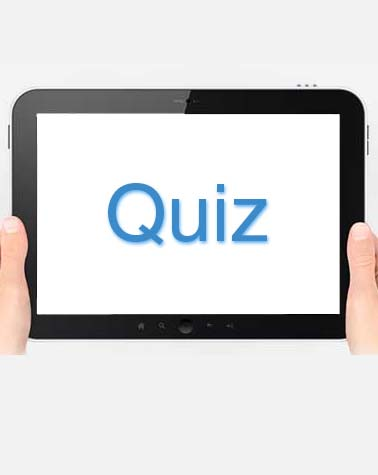# 12-1 & 12-2 Physical Science Quiz

12 Questions | Attempts: 246
ShareSettingsPhysical science is the study of non-living systems consisting of astronomy, physics, chemistry, and the Earth sciences. The 12-1 & 12-2 tests will help you best revise on this branch of science and prepare you for coming exams. Try the tests out and share your results with us. All the best!

• 1.
Which word defines the total distance traveled divided by the total time elapsed?
• A.

Velocity

• B.

Average speed

• C.

Acceleration

• D.

Motion

• 2.
Which term defines the speed of an object in a certain direction?
• A.

Velocity

• B.

Average speed

• C.

Acceleratoin

• D.

Motion

• 3.
Which term defines a change in position relative to a reference point?
• A.

Veloctiy

• B.

Average speed

• C.

Acceleration

• D.

Motion

• 4.
Which term that defines the rate at which velocity changes over time?
• A.

Motion

• B.

Acceleration

• C.

Average speed

• 5.
Which of the following best represents acceleration as presented on a graph?
• A.

Motion versus time

• B.

Distance versus time

• C.

Speed versus time

• D.

Reference point versus time

• 6.
Which of the following most closely illustrates motion by relating it to a reference point?
• A.

The hands of a clock relative to the numbers on the clock

• B.

The velocity change of a train relative to time

• C.

the speed of a bicycle relative to the direction of travel

• 7.
What causes a change in velocity?
• A.

A change in speed, a change in distance, or both

• B.

A change in speed, a change in direction, or both

• C.

A change in speed, a change in time, or both

• D.

a change in speed, a change in motion, or both

• 8.
Which of the following are the two factors used to calculate average speed?
• A.

Total distance and total time

• B.

Motion and time

• C.

velocity and time

• D.

Total acceleration and total time

• 9.
Please fill answer below. In science, a push or a pull exerted on an object is called a(n)_________.
• 10.
The SI unit for force is called_____.
• A.

Meter

• B.

Newton

• C.

Liter

• D.

Motion

• 11.
Which of the following cause an object to start moving?
• A.

Balanced forces

• B.

Either balanced or unbalanced forces

• C.

unbalanced forces

• D.

Any combination of forces

• 12.
When the net force on an object equals 0 N, the forces are which of the following?
• A.

Balanced

• B.

Unbalanced

• C.

A pull

• D.

A push

## Related TopicsBack to top
×

Wait!
Here's an interesting quiz for you.